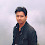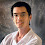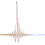### LoRa: Orthogonality

Signals which are transmitted with different chirpyness always orthogonal. Different chirp rate can be achieved by different spreading factors and/or by different bandwidths. This way LoRa symbols can by simultaneously transmitted and received on a same channel without interference.
LoRa has 6 spreading factors (SF7 - SF12) and three different bandwidths (125kHz, 250kHz & 500kHz).
Note that all the combinations of spreading factors and bandwidth are not orthogonal.

It can be simply proven by mathematics.

Symbol rate = BW / (2^SF)
and
Chirp rate = BW*(Symbol rate)
so
Chirp rate = BW* BW / (2^SF)

Case: 01 -> SF = 7, BW = 125kHz
Case: 02 -> SF = 9, BW = 250kHz

Calculate the chirp rate for both the cases. It is same, therefore both the combinations are not orthogonal and can not be used on a same channel.

Look at the below spectrogram:

Three different combinations have been plotted.

• SF: 07 & BW: 125kHz
• SF: 09 & BW: 250kHz
• SF: 11 & BW: 500kHz
Slope of the lines represents the orthogonality. Here in all three cases slope of the lines are same. Therefore all the three cases are not orthogonal.

Here is the spectrogram of orthogonal combinations:

Here the combinations are:
• SF: 10 & BW: 125kHz,
• SF: 11 & BW: 250kHz,
• SF: 12 & BW: 500kHz
Bit rate in all the three cases is different, therefore these are orthogonal combinations and can be used over same channel for the transmission.

Here is the list of orthogonal non-orthogonal combinations:

Here 'x' represents the non orthogonal combinations.

References:

If you are a research student and want to sell your work on my Blog here, please reach me on sakshama.ghosliya@gmail.com

1.i have a question sir ,do you mean that a lora module can recieve data from multiple nodes(with different bit rates ) at the same channel at the same time ? i didn't understand because in the configuration we can select only one spreading factor

1.Hello Omar, ofcourse a LoRa gateway can receive data from multiple nodes with different spreading factors given that all the conditions are same. This is how LoRaWAN can support large number of nodes. A LoRa node will not listen to all the frequencies and spreading factors at a time. Node will select a particular frequency and spreading factor to transmit and then it will open 2 receiver windows (where 2nd is optional) to receive data from network (Class A node). 1st receiver window has same frequency and SF as Tx signal where 2nd receiver window may have different SF (as it is function of Tx SF and RX1DR offset).
Notice the difference, Gateway will be continuously listening all the frequencies and all the SFs, where node will only listen to a particular frequency and SF.

2.Hi,I have a question.In the case 01 and case 02(SF=7,BW=125k and SF=9,BW=250k),you said their bit rate is same? How could it be? I cannot get the right answer. Did I misunderstand the formula ?

1.Hey Yajie Luo,

Post has been updated. It was chirp rate instead of bit rate.

3.Follow: Bit rate = SF * BW / (2^SF)
I calc in 2 cases:
SF: 07 & BW: 125kHz => Rb = 6,8 kbps
SF: 09 & BW: 250kHz => Rb= 4,3 kbps
Is it same ?

1.Hey Tran Trung,

Post has been updated. It was chirp rate instead of bit rate.

2.Thanks for your answer but according AN1200.22 LoRa™ Modulation Basics (link below), page 10, they wrote: Rc = BW. While you worte Chirp rate = BW* BW / (2^SF). What is diffrent them ? Can you explain to me ?
www.semtech.com/images/datasheet/an1200.22.pdf

3.Thanks for the comment Tran.

That is a chip rate which is defined in semtech documents. The chirp rate I defined here is different. It is the change in the frequency with respect to time. It is the slop of the chirp in above spectrogram.
Let's say for SF = 7 and BW = 125kHz
Total symbol time will be = 1.024m secs.
So the chirp rate will be = (change in frequency = 125kHz)/ (time taken = 1.024m sec) = 122.07M/sec^2

Hope it's clear now :)

4.Tks :)I understood.
I think it's same acceleration which is the rate of freq's change with respect to time.
Slope of the lines = (fmax-fmin)/Ts = BW/(2^SF/BW)= BW^2/2^SF.
And CSS uses frequency chirps with linear variation of frequency over time. The coefficient = BW^2/2^SF

4.Hi, i think that the time of one symbol with different SF is different. And the symbol duration with SF=9 is four times as the one with SF=7, but it is not in your Fig. Can you please explain it?

1.Yes of course, for SF9 symbol time will be four times of symbol time for SF7. But you should also notice BW parameter here.

2.yes, you are right. I come back to reply myself, but you reply me earlier. thanks^_^
I encounter other question. There a LoRa calculator developed by Semtech. I used it to calculate the "Time On Air" with SF=12, BW=500k, CR=1, LD off, ProgrammedPreamble=6, Header Mode off, CRC off. With these parameters, the "Syboml Time" is 8.19ms, and that's the same with my own result. when the payload length=5 bytes, the "Time on Air"=149.5ms, when i added the payload length to 6 bytes, the "Time On Air" came to 190.46ms.
(190.46-149.5) / 8.19 = 5 That means 5 symbols are added because of the extra one byte payload.
i don't know why???
i want to know how many bytes payload will occupy one symbol...

5.you can get the LoRa Calculator described in my comment here

1.2.Thanks，i've read the post, and now what's confusing me is same as that is refferred in the last comment of the same post.

assia_bMay 15
Hello BoRRoZ,

Still the 8 symbols i'm talking about aren't those of the preamble. As you see:

Time on Air = T preamble + T payload
with
and
And according to LoRa calculator, the NbPayloadSymbols is calculated with this formula:
I am looking for the meaning of those 8 symbols (red circle). In the formulas of the calculator the nPayload is independant from the 8+4.25 symb of the preamble.

i can't understand the 8 symbols (red circle) and the 4SF

6.Firstly, thanks Sakshama a lot for your generous content! This is very insightful for a beginner. And your active responses for comments are very useful too!

I have a question. Could you please clarify how a LoRaWAN gateway can respond to multiple nodes at the same time?

Is this not possible under any class of LoRaWAN? If not, how could we make this possible?

7.HI, Hope you are doing well,
For LoRa private N/W communication, where we are the concept of single Master and Multiple End Nodes. The End nodes are in listening mode with Rx and Sleep time configuration. The issue is whenever master transmit the each end node will wakeup for 2Rx+sleep time. The End node is a battery powered device. We need that the sepcific end will wake up with preamble and sync word detection. How we can resolve.